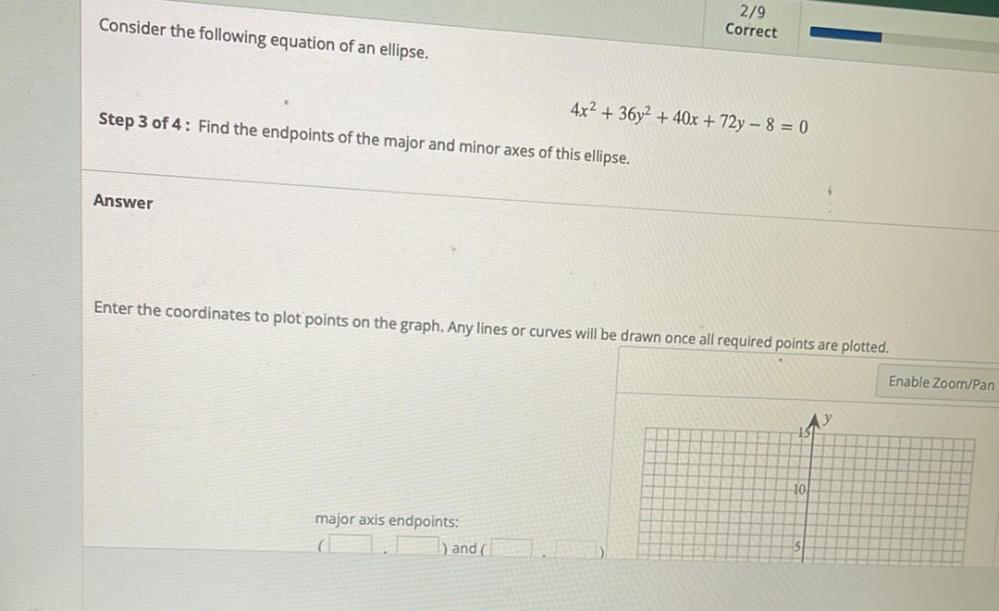Question:

# Consider the following equation of an ellipse. 2/9 Correct 4x2 + 36y2 + 40x + 72y - 8 = 0 Step 3 of 4: Find the endpoints of theConsider the following equation of an ellipse. 2/9 Correct 4x2 + 36y2 + 40x + 72y - 8 = 0 Step 3 of 4: Find the endpoints of the major and minor axes of this ellipse. Answer Enter the coordinates to plot points on the graph. Any lines or curves will be drawn once all required points are plotted. Enable Zoom/Pan 10 major axis endpoints: ) and